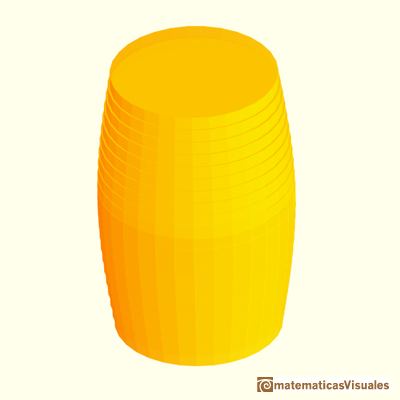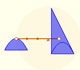Kepler: The Volume of a wine barrel

During the century before Newton and Leibniz the works of Greek mathematicians were popular, especially the work of Archimedes. Infinitesimal techniques were developed for calculating areas and volumes. Kepler was one such mathematician who contributed to these developments. His interest in calculating areas and volumes stemmed from an incident that occurred when he married for the second time. Kepler had purchased a barrel of wine for the wedding and the wine merchant?s manner of measuring the volume angered Kepler. Afterwards, he studied how to calculate areas and volumes of these kind of bodies and wrote a book about the subject. This resulted in his main contribution to the origin of integral calculus.Kepler studied Archimedes and wrote a book (published in 1615): "Nova Stereometria doliorum vinariorum" (New solid geometry of wine barrels).

It is a sistematic work on the calculation of areas and volumes by infinitesimal techniques. This work concentrates on solid of revolution, and includes determinations of the (exact or aproximate) volumes of over ninety such solids. Today we use integral calculus to solve this kind of problems.

You can see the whole book "Nova Stereometria doliorum vinariorum" in The Posner Library.

Kepler was led to develop his modification of the procedures of Archimedes. Kepler's approach in the stereometria is to dissect a given solid into an infinite number of infinitesimal pieces, or solid "indivisibles", of a size and shape convenient to the solution of the particular problem. Then he added up in some way to obtain the area or volume of the given figure.

Kepler's infinitesimal elements have the same dimension as the body he wants to measure. If he wanted to calculate an area, he added up area elements and if he wanted to calculate a volume he considered infinitesimal elements with volume.

"He thought of the volume of the cask, as of every other body, as made up of numerous thin sheets suitably ranged in layers, and considered it as the sum of the volumes of these leaves, each of which was a cylinder." (Felix Klein)Twenty years after the publication of the Stereometria doliorum of Kepler there appeared in Italy a work which rivaled it in popularity: Geometria indivisibilibus of Bonaventura Cavalieri (1635)

In this book he used what is now known as Cavalieri's Theorem: If two solids have equal altitudes, and if sections made by planes parallel to the bases and at equal distances from them are always in a given ratio, then the volumes of the solids are also in this ratio.

Cavalieri's methods differed in two significant ways from those of Kepler:

"Firstly, Cavalieri proceeded by setting up a one-to-one correspondence between the indivisible elements of two given geometical figures. If corresponding indivisibles of the two given figures had a certain (constant) ratio, he concluded that the areas or volumes of the given figures had the same ratio. Typically, the area or volume of one of the figures was known in advance, so this gave the other.

Secondly, Kepler thought of a geometrical figure as being composed of indivisibles of the same dimension. However, Cavalieri generally considered a geometrical figure to be coposed of an indefinitley large number of individibles of lower dimension. Thus he regarded an area as consisting of parallel and equidistant line segments, and a volume as consisting of parallel and equidistant plane sections.

Cavalieri made the notion of the indivisible the basis of a geometrical method of demonstration. He didn't explained precisely what he undertood by the word indivisible, which he employed to characterize the infinitesimal elements used in his method. Cavalieri conveived of a surface as made up an indefinite number of equidistant parallel lines and of a solid as composed of parallel equidistant planes, these elements being designated the indivisibles of the surface and of the volume respectively." (C.H. Edwards)

The method used by Kepler is called the method of disks. It is a mean of calculating the volume of a solid of revolution when integrating along the axis of rotation.REFERENCES

Felix Klein - Elementary Mathematics from an Advanced Standpoint. Arithmetic, Algebra, Analysis (p. 209) - Dover Publications
C.H. Edwards - The Historical Development of the Calculus (p. 99)- Springer-Verlag